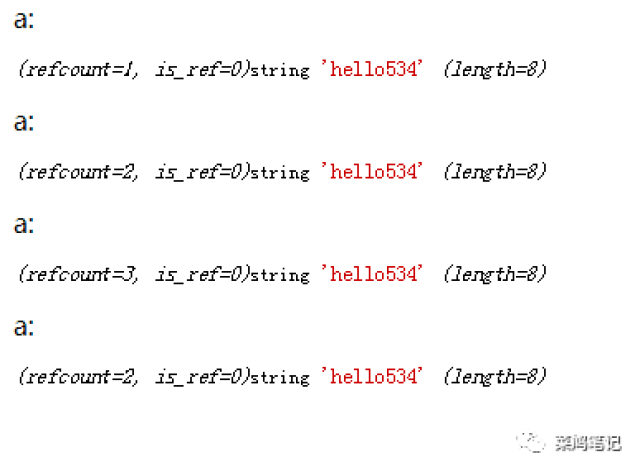# PHP的垃圾回收机制

$a = 'hello'. mt_rand( 1, 1000 ); echo xdebug_debug_zval( 'a');$b = $a; echo xdebug_debug_zval( 'a');$c = $a; echo xdebug_debug_zval( 'a'); unset($c );

echo xdebug_debug_zval( 'a');$b =$a;

$a = 123; echo$b. PHP_EOL;

// 运行结果，不用我说吧，脚趾头都知道是'hello'.mt_rand( 1, 1000 )的结果，绝对不可能是123。

<?php

$a = 'hello'. mt_rand( 1, 1000 );$b = $a; echo xdebug_debug_zval( 'a');$a = 'world'. mt_rand( 2, 2000 );

echo xdebug_debug_zval( 'a');

// 运行结果为1，其中的原理你自己应该能理顺了昂

<?php

$arr = [ 1 ];$arr[] = &$arr; unset($arr );

posted @ 2019-05-09 18:14  箫洒哥  阅读(6168)  评论(5编辑  收藏